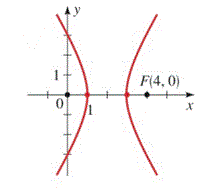Chapter 12.CT, Problem 9CT### Algebra and Trigonometry (MindTap ...

4th Edition
James Stewart + 2 others
ISBN: 9781305071742

#### Solutions

Chapter
Section### Algebra and Trigonometry (MindTap ...

4th Edition
James Stewart + 2 others
ISBN: 9781305071742
Textbook Problem

# 7-9 ■ Find an equation for the conic whose graph is shown.To determine

To find:

An equation for the conic whose graph is given.

Explanation

Given:

The graph

Approach:

Use the standard equation of the shifted ellipse (xh)2a2(yk)2b2=1.

Calculation:

In the graph values of vertices (1,0) and (3,0) are given, and values of foci (0,0) and (4,0) are given. The center will be the midpoint of vertices or foci and it will be (2,0), so h=2 and k=0. Since the distance between the center and the vertex is the value of a and the distance between the center and the focus is the value of c, so a=1 and c=2

### Still sussing out bartleby?

Check out a sample textbook solution.

See a sample solution

#### The Solution to Your Study Problems

Bartleby provides explanations to thousands of textbook problems written by our experts, many with advanced degrees!

Get Started